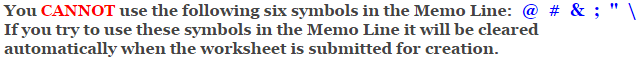# Calculus

## Mean Value Theorem Worksheets

These Calculus Worksheets will produce problems that involve finding a value that satisfies the mean value theorem, given a function and a domain.
The student will be given a function and a domain and will be asked to find the values that satisfy the mean value theorem.
You may select the number of problems, and the types of functions to include.

These Mean Value Theorem Worksheets are a great resource for Definite Integration.

### Language for the Mean Value Theorem Worksheets

 English German Albanian Spanish Swedish Italian French Turkish Polish Norwegian

### Memo Line for the Mean Value Theorem Worksheets

You may enter a message or special instruction that will appear on the bottom left corner of the Mean Value Theorem Worksheets.### Mean Value Theorem Worksheets Answer Page

Now you are ready to create your Mean Value Theorem Worksheets by pressing the Create Button.

 Recommended Videos

If You Experience Display Problems with Your Math Worksheet